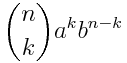{{announcement.body}}
{{announcement.title}}

# The Rise and Fall of Binomial Coefficients

DZone 's Guide to

# The Rise and Fall of Binomial Coefficients

· Big Data Zone ·
Free Resource

Comment (0)

Save
{{ articles.views | formatCount}} Views

When you expand (x + y)n, the coefficients increase then decrease. The largest coefficient is in the middle if n is even; it’s the two in the middle if n is odd. For example, the coefficients for (1 +x)4 are 1, 4, 6, 4, 1 and the coefficients for (1 + x)5 are 1, 5, 10, 10, 5, 1.

More generally, if a > 0 and b > 0, the coefficients of (ax + by)n can only have one maximum. They may increase, or decrease, or change direction once, but they cannot wiggle more than that. They can’t, for example, increase, decrease, then increase again.

Here’s a proof. The coefficients areTo show that the coefficients are unimodal as a function of k, we’ll show that their logarithms are unimodal. And we’ll do that by showing that they are samples from a concave function.

The log of the kth coefficient is

log Γ(n+1) – log Γ(k+1) – log Γ(n-k+1) + k log a + (n-k) log b.

As a function of k, the terms

log Γ(n+1) + k log a + (n-k) log b

form an affine function. The function log Γis convex, so -log Γis concave. The composition of a concave function with an affine function is concave, so – log Γ(k+1) and – log Γ(n-k+1) are concave functions of k. The sum of concave functions is concave. And the sum of a concave function with an affine function is concave. So binomial coefficients are log-concave and they can only have one maximum.

(The fact log Γ(z) is a convex is the easy direction of the Bohr-Mollerup theorem. The harder direction is that Γ(z) is the only way to extend factorials to all reals that is log-convex.)

Topics:

Comment (0)

Save
{{ articles.views | formatCount}} Views

Published at DZone with permission of John Cook , DZone MVB. See the original article here.

Opinions expressed by DZone contributors are their own.